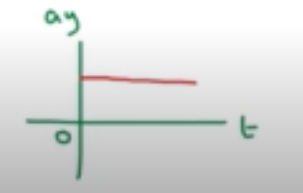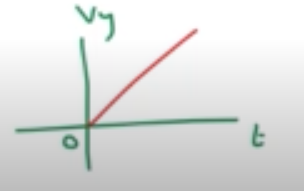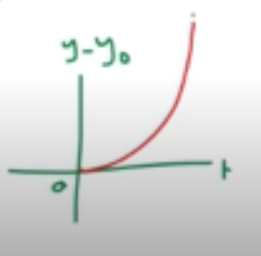Need Help?

Subscribe to Physics 1

###### \${selected_topic_name}
• Notes
• Comments & Questions

$\begin{array}{l}{\text { (a) If a flea can jump straight up to a height of 0.440 } \mathrm{m} \text { , }} \\ {\text { what is its initial speed as it leaves the ground? (b) How long is it }} \\ {\text { in the air? }}\end{array}$

$V_{y}= 0, \quad y-y_{0}=0.440 \mathrm{m}, \quad a_ y=-9.8 \mathrm{m} / \mathrm{s}^{2}$

$V_{y}^{2}=v_{0 y}^{2}+2 a y\left(y-y_{0}\right)$

$\rightarrow V_{0y}=\sqrt{-2 a y\left(y-y_{0}\right)}=\sqrt{-2(-9.8)(0.44)}=2.94 \mathrm{m} / \mathrm{s}$

$y-y_{0}=0 \longrightarrow V_{0 y}=+2.94 \mathrm{m} / \mathrm{s}, \quad a y=-9.8 \mathrm{m} / \mathrm{s}^{2}$

$y- y_{0}=v_{0 y} t+\frac{1}{2} a yt^{2} \rightarrow t=-\frac{2 v_{0 y}}{a y}=\frac{-2(2.94)}{-9.8}=0.6s$

$\begin{array}{l}{\text { A small rock is thrown vertically upward with a speed of } 18 \mathrm{m} / \mathrm{s} \text { from the edge of the roof of a } 30.0 \mathrm{m} \text { -tall building. }} \\ {\text { The rock doess't hit the building on its way back down and lands in the street below. Air resistance can be neglect }} \\ {\text { (a) What is the speed of the rock just before it hits the street? (b) How much time elapses from when the rock is }} \\ {\text { thrown until it hits the street? }}\end{array}$

$y-y_{0}=-30 m, \quad V_{0y}=18 m / s \ , a y=-9.8 \mathrm{m} / \mathrm{s}^{2}$

$v_{y}=\sqrt{v_{0y}^{2}+2 a_{y}\left(y-y_{0}\right)}=\sqrt{\left.(18)^{2}+2(9.8)(-30\right)}=-30.2 \mathrm{m} / \mathrm{s}$

$V_{y}=v_{0 y}+a_{y} t \rightarrow t=\frac{v_{y}-v_{0y}}{a_{y}}=\frac{-30.3-18}{-9.8}=4.92 \mathrm{s}$

$\begin{array}{l}{\text { A brick is dropped (zero initial speed) firom the roof of a builiding. The brick }} \\ {\text { strikes the groud in } 2.50 \text { s. is the building? (b) What is the magnitude of the }} \\ {\text { brick's velocity just before it reaches the ground? (c) Sketch ay-t, vy-t and } y-t} \\ {\text { graphs for the motion of the brick. }}\end{array}$

$V_{0 y}=0, \quad t=2.5 s \quad, \quad a_{y}=9.8 \mathrm{m} / \mathrm{s}^{2},$

$y - y_{0}= v_{0 y} t+\frac{1}{2} a _{y} t^{2}=\frac{1}{2}(9.8)(2.5)^{2}=30.6 \mathrm{m}$

$V_ y=V_{0y}+a y t=0+9.8(2.5)=24.5 \mathrm{m} / \mathrm{s}$No comments yet